# How to Create a Pivot Table in Python using Pandas?

• Last Updated : 28 Jul, 2020

Pivot table is a statistical table that summarizes a substantial table like big datasets. It is part of data processing. This summary in pivot tables may include mean, median, sum, or other statistical terms. Pivot tables are originally associated with MS Excel but we can create a pivot table in Python using Pandas using the dataframe.pivot() method.

Syntax : dataframe.pivot(self, index=None, columns=None, values=None, aggfunc)

Parameters –
index: Column for making new frame’s index.
columns: Column for new frame’s columns.
values: Column(s) for populating new frame’s values.
aggfunc: function, list of functions, dict, default numpy.mean

Example 1:
Let’s first create a dataframe that includes Sales of Fruits.

 `# importing pandas``import` `pandas as pd`` ` `# creating dataframe``df ``=` `pd.DataFrame({``'Product'` `: [``'Carrots'``, ``'Broccoli'``, ``'Banana'``, ``'Banana'``,``                                ``'Beans'``, ``'Orange'``, ``'Broccoli'``, ``'Banana'``],``                   ``'Category'` `: [``'Vegetable'``, ``'Vegetable'``, ``'Fruit'``, ``'Fruit'``,``                                 ``'Vegetable'``, ``'Fruit'``, ``'Vegetable'``, ``'Fruit'``],``                   ``'Quantity'` `: [``8``, ``5``, ``3``, ``4``, ``5``, ``9``, ``11``, ``8``],``                   ``'Amount'` `: [``270``, ``239``, ``617``, ``384``, ``626``, ``610``, ``62``, ``90``]})``df`

Output: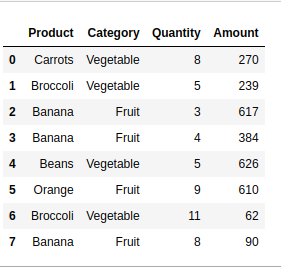Get the total sales of each product

 `# creating pivot table of total sales``# product-wise aggfunc = 'sum' will ``# allow you to obtain the sum of sales``# each product``pivot ``=` `df.pivot_table(index ``=``[``'Product'``],``                       ``values ``=``[``'Amount'``],``                       ``aggfunc ``=``'sum'``)``print``(pivot)`

Output: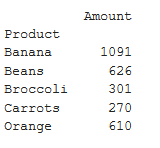Get the total sales of each category

 `# creating pivot table of total ``# sales category-wise aggfunc = 'sum'``# will allow you to obtain the sum of``# sales each product``pivot ``=` `df.pivot_table(index ``=``[``'Category'``], ``                       ``values ``=``[``'Amount'``], ``                       ``aggfunc ``=``'sum'``)``print``(pivot)`

Output: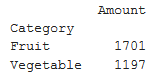Get the total sales of by category and product both

 `# creating pivot table of sales``# by product and category both``# aggfunc = 'sum' will allow you``# to obtain the sum of sales each``# product``pivot ``=` `df.pivot_table(index ``=``[``'Product'``, ``'Category'``], ``                       ``values ``=``[``'Amount'``], aggfunc ``=``'sum'``)``print` `(pivot)`

Output –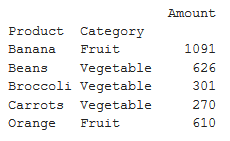Get the Mean, Median, Minimum sale by category

 `# creating pivot table of Mean, Median,``# Minimum sale by category aggfunc = {'median',``# 'mean', 'min'} will get median, mean and ``# minimum of sales respectively``pivot ``=` `df.pivot_table(index ``=``[``'Category'``], values ``=``[``'Amount'``], ``                       ``aggfunc ``=``{``'median'``, ``'mean'``, ``'min'``})``print` `(pivot)`

Output –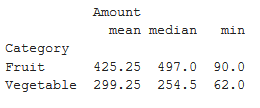Get the Mean, Median, Minimum sale by product

 `# creating pivot table of Mean, Median,``# Minimum sale by product aggfunc = {'median',``# 'mean', 'min'} will get median, mean and``# minimum of sales respectively``pivot ``=` `df.pivot_table(index ``=``[``'Product'``], values ``=``[``'Amount'``],``                       ``aggfunc ``=``{``'median'``, ``'mean'``, ``'min'``})``print` `(pivot)`

Output: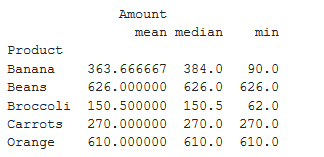My Personal Notes arrow_drop_up